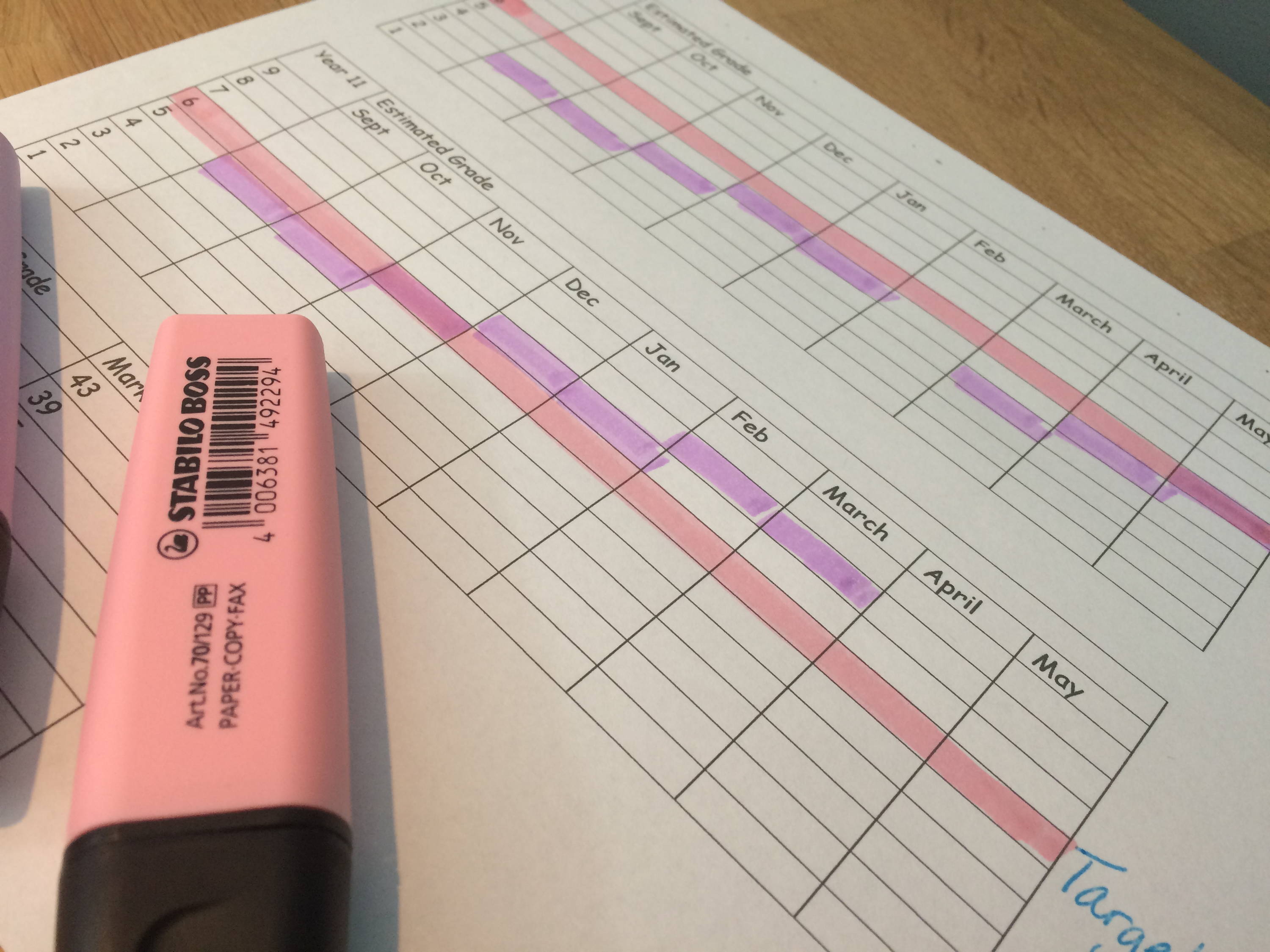Liquid error: Could not find asset snippets/loyaltylion.liquid

This is in the process of being moved to a new site, if you have a membership to this site you will be given access to the new one

• Number
• Algebra
• Ratio, proportion and rates of change
• Geometry and measure
•  Probability and Statistics

# Topics covered

Subtracting Fractions

Multiples

Prime numbers

Square, Cube & Roots

Percentages (Non-Calculator)

Converting decimals to fractions

Rounding to decimal place

Rounding to significant figures

Convert from standard form

Convert to standard form

Estimations

Estimating Roots

Bonds

BODMAS

Signs

Indices

Multiplying Fractions

Surds

Dividing Fractions

Ordering numbers

Ordering Fractions

Ordering Decimals

Square numbers

Square roots

Cube numbers

Cube roots

# Topics covered...

Expand Double Brackets

Solving inequalities

Direct proportion

Midpoints

Laws of indices

Special Sequences

Geometric Sequences

Linear Graphs (One Variable)

Parallel and Perpendicular

Coordinates

Collecting like terms

Putting numbers in algebraic expression

Solving Equations

# Topics covered...

Interests

Percentages (With Calculator)

Simplifying Ratio

Sharing into a ratio

Unitary Ratio

Linear Sequences

Comparing using percentages

Reverse percentages

Indirect proportion

# Topics covered...

Angles in Triangles

Pythagoras Theorem

Trigonometry Sides

Trigonometry Angles

Sine Rule

Cosine Rule

Areas in Triangles

Trigonometry Areas in Triangles

Basic Angle Facts

Parallel Angle Facts

Inferior/Exterior

Area and circumference of circles

Area of squares and rectangles

Perimeter

Reverse Area

Simultaneous Linear Equations

# Topics covered...

Averages from a list

Probability of events

Probability (Tables)

Tree Diagrams

Venn Diagrams

Pie Charts

Bar Charts

If you try these quizzes every month (or every other month) from now until your exams you should know the content really, really well. In the revision guide highlight your target grade in one colour, and every time you have completed a quiz (or a test in school) mark that on the table, so you can see how you are improving over time!

Please note the grade boundaries used in this book are estimates, based on best data, this does not guarantee you this grade. You still have to work hard!